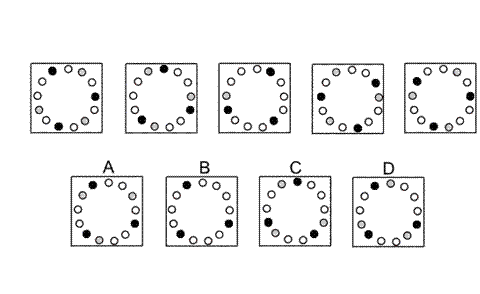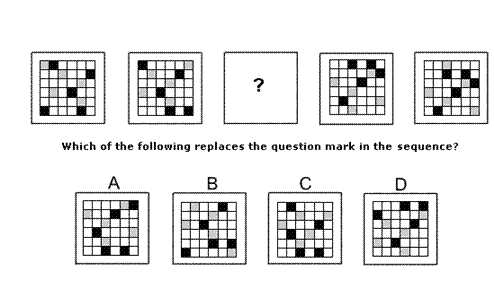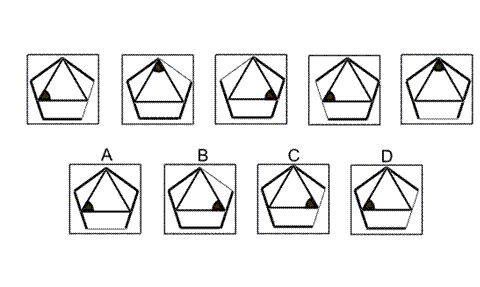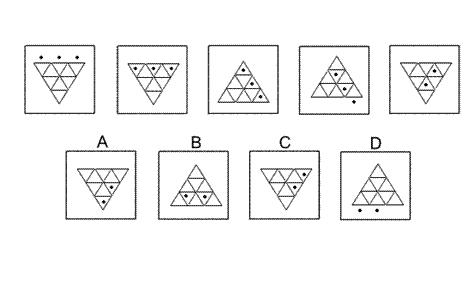Aptitude Tests 4 Me

Non Verbal Logic

Detailed solution

16.Solution: B Explanation: In this question, the black and grey dots move around the circle of dots. The black dots move one place clockwise each time. The grey dots move two places anticlockwise each time. When a grey and a black dot occupy the same position, the grey is obscured by the black and only the black dot is visible. For the next diagram of the sequence, all three grey dots are obscured by black dots. The correct answer, therefore, is B.

17.Solution: A Explanation: In this question, there are three shapes - an asterisk, a square and a dot. Each shape has its own rule to follow. The asterisk rotates around the inside of the hexagon by 120° clockwise each time. The square moves across the horizontal axis of symmetry of the hexagon from one vertex to the opposite vertex, then back again. Its colour changes from grey to black to white, back to grey etc. The dot moves up and down the vertical axis of symmetry of the hexagon, occupying three positions - two opposite edges and the centre of the hexagon. Its colour alternates between black and white. Following all these rules, the correct answer is A.

18.Solution: D Explanation: In this question there are two rules that are applied alternately. The first rule is that all squares are moved one place to the left, except those in the left column which are moved to the right column. The second rule is that all squares are moved one place down, except those in the bottom row which are moved to the top row. To find the missing diagram of the sequence, the second rule should be applied, and the correct answer is D.

19.Solution: C Explanation: In this question there is an equilateral triangle inside a regular pentagon. Each shape follows its own rule. The equilateral triangle rotates by 120° clockwise each time. Following this rule, the correct answer could be B or C. The regular pentagon rotates by 72° anticlockwise each time. Following this rule, the correct answer could be C or D. When both rules are applied simultaneously, therefore, the correct answer must be C.

20.Solution: A Explanation: In this question there are three balls that move into, around and out of a grid of equilateral triangles. There are two rules to follow that are applied alternately. The first rule is that the balls drop down through a horizontal edge of a triangle. If a ball drops down outside the grid, it is lost and doesn't appear in the next diagram of the sequence. The second rule is that the triangular grid rotates through 120° clockwise. For the next diagram of the sequence, the first rule should be applied and the correct answer is A.

 1 2 3 4 5 6 7 8 9 10 11 12 13 14 15 16 17 18 19 20 21 22 23 24 25 26 27 28 29 30 31 32 33 34 35 36 37 38 39 40 41 42 43 44 45 46 47 48 49 50 51 52 53 54 55 56 57 58 59 60 61 62 63 64 65 66 67 68 69 70 71 72 73 74 75 76 77 78 79 80 81 82 83 84 85 86 87 88Passage ReadingVerbal LogicNon Verbal LogicNumerical LogicData InterpretationReasoningAnalytical AbilityBasic NumeracyAbout UsContactPrivacy PolicyMajor TestsFAQ